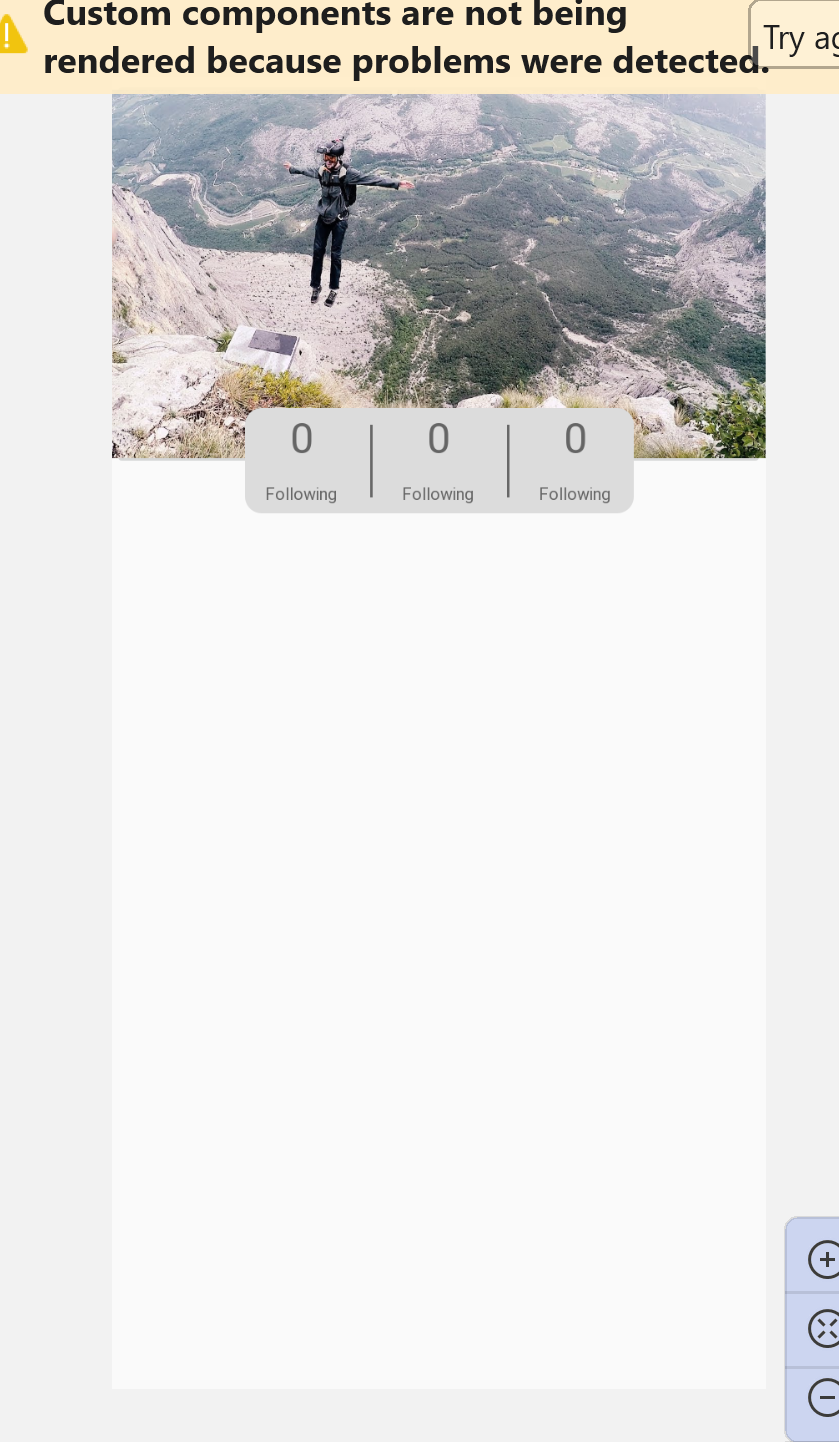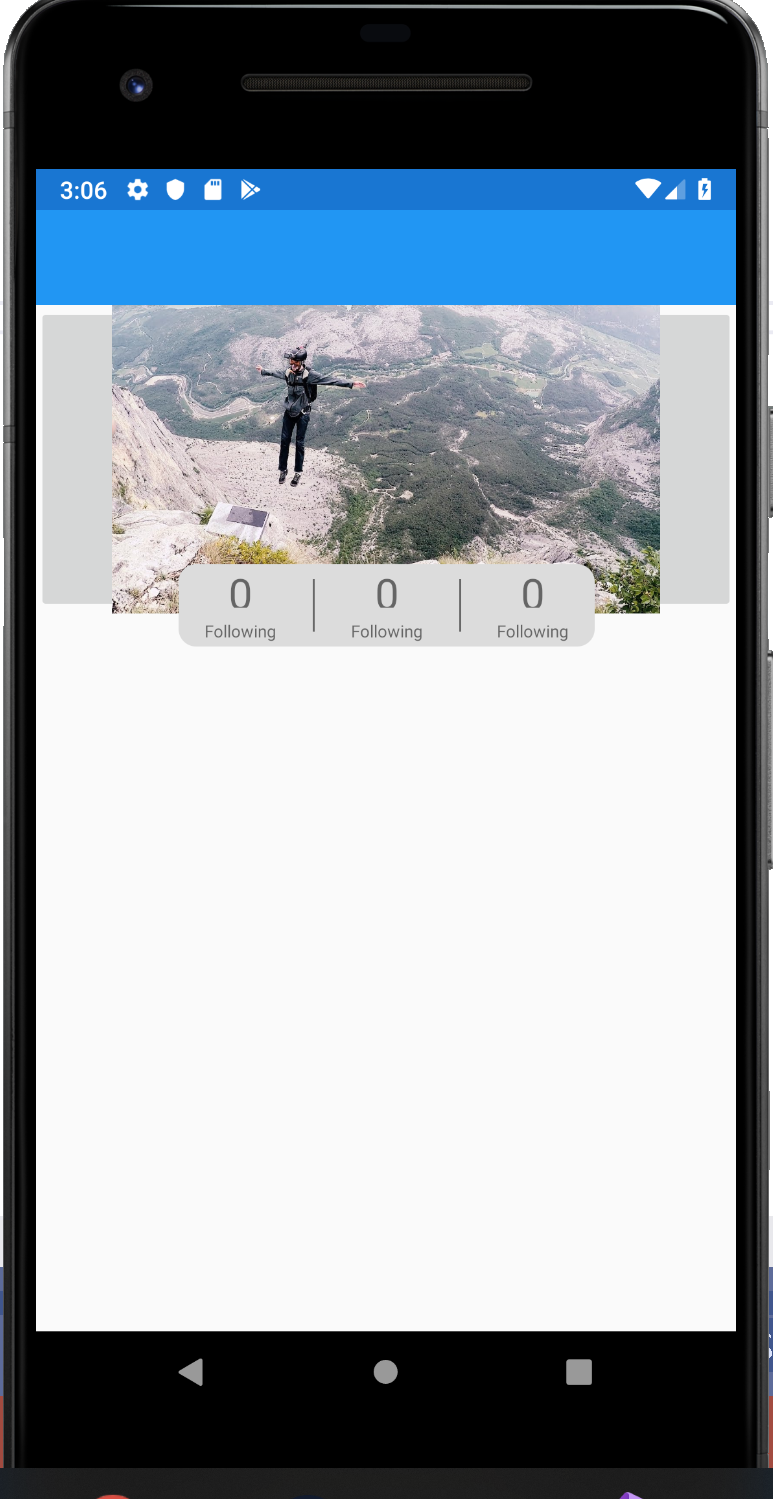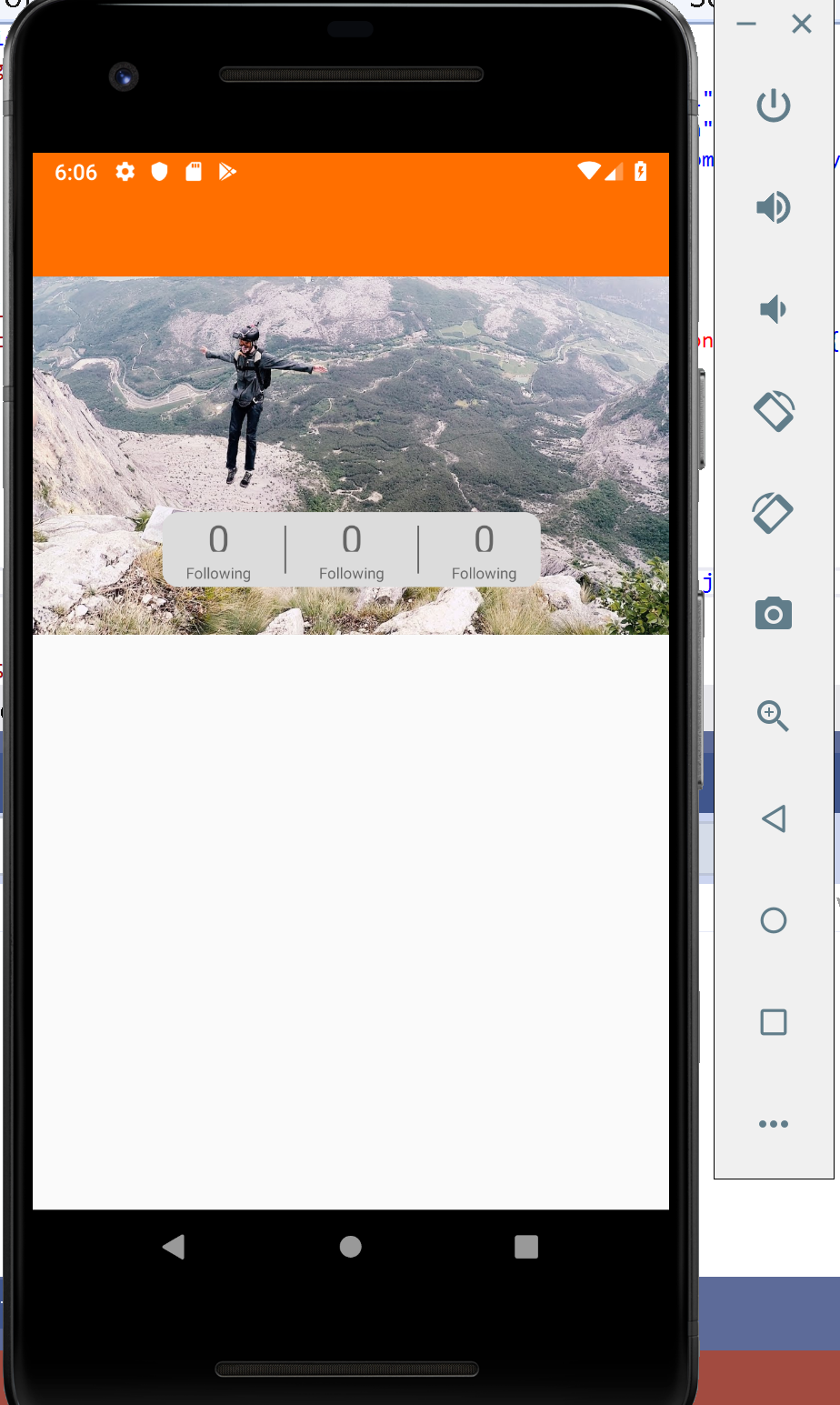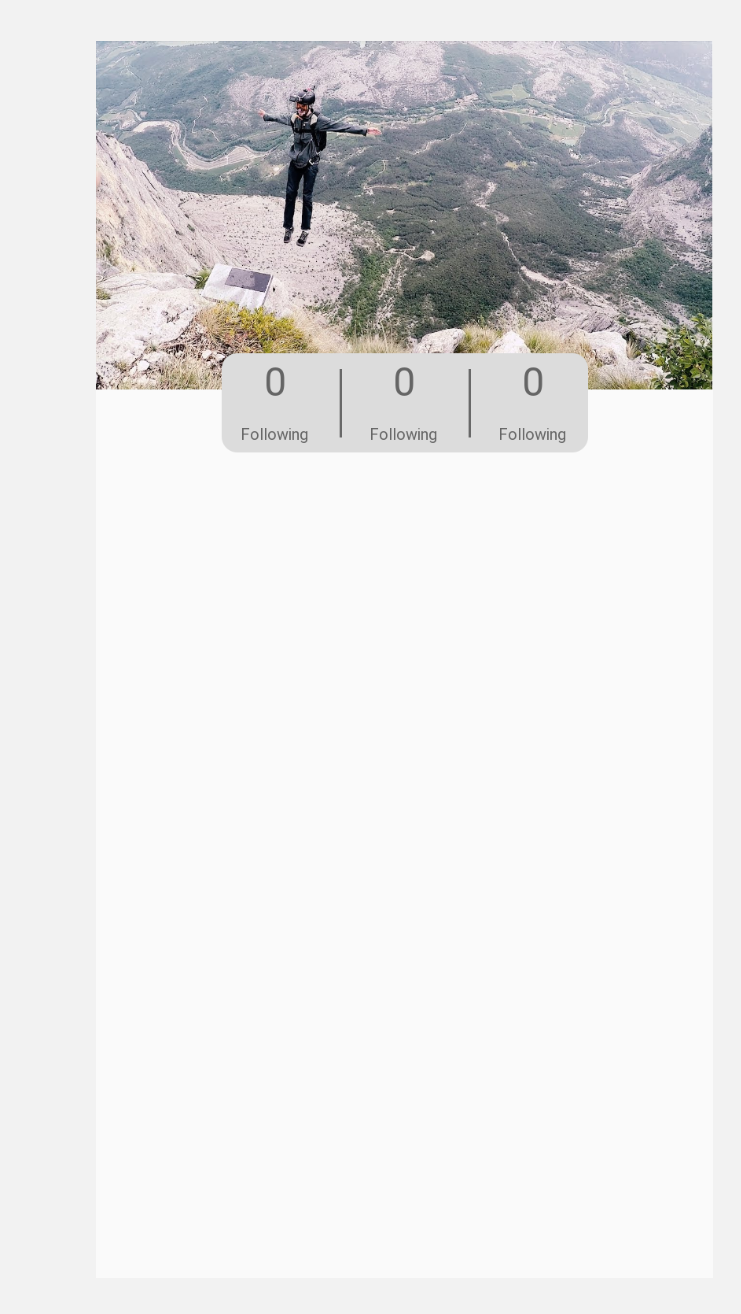# how to properly place view for all deviced

edited November 2019

im having a problem placing a box view exactly on the middle of a line without it being cut off i will add picture so this makes sense and also imageview has gray rectangle behind it in the emulator```    <Grid RelativeLayout.HeightConstraint="{ConstraintExpression
Type=RelativeToParent,
Property=Height,
Factor=.08}"
RelativeLayout.WidthConstraint="{ConstraintExpression
Type=RelativeToParent,
Property=Width,
Factor=1}"
RelativeLayout.YConstraint="{ConstraintExpression
Type=RelativeToView,
ElementName=profileImage,
Property=Height,
Factor=.84

}">
<Grid.RowDefinitions>
<RowDefinition Height="1*"/>

</Grid.RowDefinitions>
<Grid.ColumnDefinitions>
<ColumnDefinition Width=".2*"/>
<ColumnDefinition Width=".2*"/>
<ColumnDefinition Width=".2*"/>
<ColumnDefinition Width=".2*"/>
<ColumnDefinition Width=".2*"/>
</Grid.ColumnDefinitions>
<BoxView Grid.Row="0" Grid.Column="0"/>
<Grid>
<Grid.RowDefinitions>
<RowDefinition Height="1*"/>
</Grid.RowDefinitions>
<Grid.ColumnDefinitions>
<ColumnDefinition Width=".33*"/>
<ColumnDefinition Width=".005*"/>
<ColumnDefinition Width=".33*"/>
<ColumnDefinition Width=".005*"/>
<ColumnDefinition Width=".33*"/>
</Grid.ColumnDefinitions>
<Grid Grid.Row="0" Grid.Column="0">
<Grid.RowDefinitions>
<RowDefinition Height=".6*"/>
<RowDefinition Height=".4*"/>
</Grid.RowDefinitions>
<Grid.ColumnDefinitions>
<ColumnDefinition Width="1*"/>
</Grid.ColumnDefinitions>
<Label Grid.Row="0" Grid.Column="0" Text="0" FontSize="25" HorizontalOptions="Center" VerticalOptions="Center"/>
<Label Grid.Row="1" Grid.Column="0"  Text="Following" FontSize="10" HorizontalOptions="Center" VerticalOptions="Center"/>
</Grid>
<Grid Grid.Row="0" Grid.Column="1">
<Grid.RowDefinitions>
<RowDefinition Height=".075*"/>
<RowDefinition Height=".85*"/>
<RowDefinition Height=".075*"/>
</Grid.RowDefinitions>
<Grid.ColumnDefinitions>
<ColumnDefinition Width="1*"/>
</Grid.ColumnDefinitions>
<BoxView Grid.Row="0" Grid.Column="0"/>
<BoxView Grid.Row="1" Grid.Column="0" BackgroundColor="#686868" />
<BoxView Grid.Row="2" Grid.Column="0"/>
</Grid>
<Grid  Grid.Row="0" Grid.Column="2" >
<Grid.RowDefinitions>
<RowDefinition Height=".6*"/>
<RowDefinition Height=".4*"/>
</Grid.RowDefinitions>
<Grid.ColumnDefinitions>
<ColumnDefinition Width="1*"/>
</Grid.ColumnDefinitions>
<Label Grid.Row="0" Grid.Column="0" Text="0" FontSize="25" HorizontalOptions="Center" VerticalOptions="Center"/>
<Label Grid.Row="1" Grid.Column="0"  Text="Following" FontSize="10" HorizontalOptions="Center" VerticalOptions="Center"/>
</Grid>
<Grid Grid.Row="0" Grid.Column="3" >
<Grid.RowDefinitions>
<RowDefinition Height=".075*"/>
<RowDefinition Height=".85*"/>
<RowDefinition Height=".075*"/>
</Grid.RowDefinitions>
<Grid.ColumnDefinitions>
<ColumnDefinition Width="1*"/>
</Grid.ColumnDefinitions>
<BoxView Grid.Row="0" Grid.Column="0"/>
<BoxView Grid.Row="1" Grid.Column="0" BackgroundColor="#686868"/>
<BoxView Grid.Row="2" Grid.Column="0"/>
</Grid>
<Grid  Grid.Row="0" Grid.Column="4">
<Grid.RowDefinitions>
<RowDefinition Height=".6*"/>
<RowDefinition Height=".4*"/>
</Grid.RowDefinitions>
<Grid.ColumnDefinitions>
<ColumnDefinition Width="1*"/>
</Grid.ColumnDefinitions>
<Label Grid.Row="0" Grid.Column="0" Text="0" FontSize="25" HorizontalOptions="Center" VerticalOptions="Center"/>
<Label Grid.Row="1" Grid.Column="0"  Text="Following" FontSize="10" HorizontalOptions="Center" VerticalOptions="Center"/>
</Grid>

</Grid>
</Frame>

<BoxView Grid.Row="0" Grid.Column="4"/>

</Grid>

</RelativeLayout>
```

• Do you mean set the image to fill the Button. If so, try using StackLayout instead of ImageButton, then put the image into the layout. For the tap feature, you can add a tap gesture on the layout.

```<StackLayout
x:Name="profileImage"
...>
<Image Aspect="AspectFit" Source="grid_"/>
</StackLayout>
```
• edited November 2019

i did it and it solved the image problem but now thw grid doesnt line up with the image and the 0's are being cut off a bit@Jarvan said:
Do you mean set the image to fill the Button. If so, try using StackLayout instead of ImageButton, then put the image into the layout. For the tap feature, you can add a tap gesture on the layout.

```<StackLayout
x:Name="profileImage"
...>
<Image Aspect="AspectFit" Source="grid_"/>
</StackLayout>
```

new code

```    </StackLayout>

<Grid RelativeLayout.HeightConstraint="{ConstraintExpression
Type=RelativeToParent,
Property=Height,
Factor=.08}"
RelativeLayout.WidthConstraint="{ConstraintExpression
Type=RelativeToParent,
Property=Width,
Factor=1}"
RelativeLayout.YConstraint="{ConstraintExpression
Type=RelativeToView,
ElementName=profileImage,
Property=Height,
Factor=.84

}">
<Grid.RowDefinitions>
<RowDefinition Height="1*"/>

</Grid.RowDefinitions>
<Grid.ColumnDefinitions>
<ColumnDefinition Width=".2*"/>
<ColumnDefinition Width=".2*"/>
<ColumnDefinition Width=".2*"/>
<ColumnDefinition Width=".2*"/>
<ColumnDefinition Width=".2*"/>
</Grid.ColumnDefinitions>
<BoxView Grid.Row="0" Grid.Column="0"/>
<Grid>
<Grid.RowDefinitions>
<RowDefinition Height="1*"/>
</Grid.RowDefinitions>
<Grid.ColumnDefinitions>
<ColumnDefinition Width=".33*"/>
<ColumnDefinition Width=".005*"/>
<ColumnDefinition Width=".33*"/>
<ColumnDefinition Width=".005*"/>
<ColumnDefinition Width=".33*"/>
</Grid.ColumnDefinitions>
<Grid Grid.Row="0" Grid.Column="0">
<Grid.RowDefinitions>
<RowDefinition Height=".6*"/>
<RowDefinition Height=".4*"/>
</Grid.RowDefinitions>
<Grid.ColumnDefinitions>
<ColumnDefinition Width="1*"/>
</Grid.ColumnDefinitions>
<Label Grid.Row="0" Grid.Column="0" Text="0" FontSize="25" HorizontalOptions="Center" VerticalOptions="Center"/>
<Label Grid.Row="1" Grid.Column="0"  Text="Following" FontSize="10" HorizontalOptions="Center" VerticalOptions="Center"/>
</Grid>
<Grid Grid.Row="0" Grid.Column="1">
<Grid.RowDefinitions>
<RowDefinition Height=".075*"/>
<RowDefinition Height=".85*"/>
<RowDefinition Height=".075*"/>
</Grid.RowDefinitions>
<Grid.ColumnDefinitions>
<ColumnDefinition Width="1*"/>
</Grid.ColumnDefinitions>
<BoxView Grid.Row="0" Grid.Column="0"/>
<BoxView Grid.Row="1" Grid.Column="0" BackgroundColor="#686868" />
<BoxView Grid.Row="2" Grid.Column="0"/>
</Grid>
<Grid  Grid.Row="0" Grid.Column="2" >
<Grid.RowDefinitions>
<RowDefinition Height=".6*"/>
<RowDefinition Height=".4*"/>
</Grid.RowDefinitions>
<Grid.ColumnDefinitions>
<ColumnDefinition Width="1*"/>
</Grid.ColumnDefinitions>
<Label Grid.Row="0" Grid.Column="0" Text="0" FontSize="25" HorizontalOptions="Center" VerticalOptions="Center"/>
<Label Grid.Row="1" Grid.Column="0"  Text="Following" FontSize="10" HorizontalOptions="Center" VerticalOptions="Center"/>
</Grid>
<Grid Grid.Row="0" Grid.Column="3" >
<Grid.RowDefinitions>
<RowDefinition Height=".075*"/>
<RowDefinition Height=".85*"/>
<RowDefinition Height=".075*"/>
</Grid.RowDefinitions>
<Grid.ColumnDefinitions>
<ColumnDefinition Width="1*"/>
</Grid.ColumnDefinitions>
<BoxView Grid.Row="0" Grid.Column="0"/>
<BoxView Grid.Row="1" Grid.Column="0" BackgroundColor="#686868"/>
<BoxView Grid.Row="2" Grid.Column="0"/>
</Grid>
<Grid  Grid.Row="0" Grid.Column="4">
<Grid.RowDefinitions>
<RowDefinition Height=".6*"/>
<RowDefinition Height=".4*"/>
</Grid.RowDefinitions>
<Grid.ColumnDefinitions>
<ColumnDefinition Width="1*"/>
</Grid.ColumnDefinitions>
<Label Grid.Row="0" Grid.Column="0" Text="0" FontSize="25" HorizontalOptions="Center" VerticalOptions="Center"/>
<Label Grid.Row="1" Grid.Column="0"  Text="Following" FontSize="10" HorizontalOptions="Center" VerticalOptions="Center"/>
</Grid>

</Grid>
</Frame>

<BoxView Grid.Row="0" Grid.Column="4"/>

</Grid>

</RelativeLayout>
```

• Reset `RelativeLayout.WidthConstraint` and `RelativeLayout.HeightConstraint` of stacklaout. Or you can change the value of image's HeightRequest.

• edited November 2019

but in the designer it shows it being fineand the reason i say this is it seems to change location depending on the display and i want the middle of the gray box locked on to the bottom of the image on all displays no matter the size of image or display

• edited November 2019

Maybe the two devices are not the same. Try to select the corresponding type device with your emulator on previewer or use the same emulator as the previewer shown.

• edited November 2019

@Jarvan said:
Maybe the two devices are not the same. Try to select the corresponding type device with your emulator on previewer or use the same emulator as the previewer shown.

i plan to release this app someday on a wide range on devices so i need to figure out how to in the code if there is a way
thanks for the help

• @Jarvan said:

release this app someday on a wide range on devices

If image‘s is set to a specific value like 'width=50', it will show different effects on different devices. But if you are setting the scale or filling the screen, there won't be much difference.

how to properly place view for all deviced

Sorry for my mistake, try to set ImageButton's Aspect to AspectFill directly. The image will fill the ImageButton. You may need to adjust the aspect ratio of the image to adapt the button.

```<ImageButton
x:Name="profileImage"
Aspect="AspectFill"
Source="1120"
RelativeLayout.WidthConstraint="{...}"
RelativeLayout.HeightConstraint="{...}"/>
```

thank you this solved my problem and is proportionally the same across multiple emulators